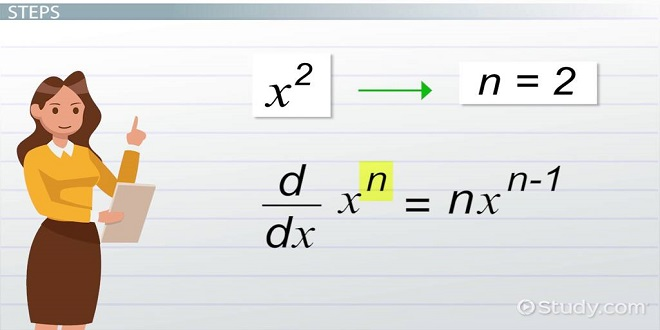# Learn Easy Methods to Solve Calculus – Derivative Formulas

Maths is a very interesting topic. There are many simple and some complex topics in it. Have you heard of calculus in Maths? Let’s learn about it.

Let us say that Riya is traveling some distance “p” km in time “t” seconds from city “A”. Now, if we ask how fast or slow Riya reached another city “B”, how do we determine that? Voila! There is an interesting method that we can apply in maths to find the speed, and that is derivatives. So, here, “p” is the distance covered in time “t” is written as dp/dt.

Also, dp/dt = v (velocity)

Or,

dp/dt = f (p); this is how we write derivative for the speed.

Now, if we say how much Riya’s speed varied in given intervals of time, then we write it in the following manner:

dv/dt = a (acceleration)

Or,

d2p/dt2 = a

This is how we write the double derivative for finding the acceleration.

Now, let us go through a story of Neha running after her school bus to understand calculus with derivative formulas.

## A Story of Neha Running after her School Bus

One day, Neha was running after her school bus. Unfortunately, she got tired and found that the bus was 2 km ahead of the point, where she rested. The next day, she found that the same bus was 4 km ahead, the next day, 8 km, and so on…..

Now, what do we find here? Well, if we say “q” = 2 km, “q2” = 4 km, and “q3” = 8 km,….,  qn = n km. So, if we want to find the derivative of this equation with respect to “q” itself, how do we do? Well, below is the following method:

d (qn) /dq   =  n. qn – 1

This is a Power formula.

## Derivative of a Theta Family

Today, six members of the theta family (Sin , Cos , Tan , Cot , Cosec, Sec ) are going to their favorite locations.

Sin went to its favorite resort through the ABC path and Cos came back through the same path, while Tan , Cot , Cosec , and Sec went on their favorite locations, respectively. While going to their destination, they all wanted to know the derivative of each. The family members had the following derivative:

Sin while going through the derivative, it reached to city Cos . When Cos went back to her hometown, she traveled the opposite direction, i.e., negative of the original path, it reached – Sin .

• d (Sin )/d = Cos
• d (Cos )/d = – Sin

Similarly, Tan , Cot , Cosec , and Sec made their way to reach their specific locations in the following manner:

• d (Tan )/d = Sec2
• d (Cot )/d = –  Cosec2
• d (Cosec )/d = – Cosec Cot
• d (Sec )/d = Sec Tan

Now, let us see basic derivative formula rules.

Basic Derivative Formulas with Rules

• If we have to find the derivative of a constant:

d (c)/dp = 0

Please note that the derivative of a constant is always zero.

• d (n)/dn = 1
• Constant multiple Rule:

d [c f (p)]/dp = c f’ (p)

• Sum Rule:

d/dp [f (p) + g (p)] = f’ (p) + g’ (p)

• Difference Rule:

d/dp [f (p) – g (p)] = f’ (p) – g’ (p)

• Product Rule:

d/dp [f (p) * (g (p)] = f’ (p). g (p) + f (p). G (p)’

• Quotient Rule:

d[f (p) /g (p)]/dp  =  [g (p). f’ (p) – f (p). g’ (p)]/[g (p)]

• Chain Rule:

d[f (g (p)]/dp  =  f’ [g (p)] g’ (p)

## Solved Example on Derivative Formula

Solve f (n)  =  2 n3 + 300/n2 + 4 at n = 2.

Solution: d f (n)/dn = 2 n3 + 300n– 2 + 4

=> 3 .  2n3 – 1 + (- 2) 300 n– 2 – 1  + 4

=> 3 . 2n2 + (- 2) 300 n– 3  + 4

Now, putting n = 2:

=> 3 . 2 (2)2 + (- 2) 300 (2)– 3  + 4

=> 24 – [600 * (⅛)] + 4

=> 24 – 75 + 4

=> 47

Therefore, the derivative of the above question at n = 2 is 47.

In mathematics, we must note that calculus-derivative formulas have a great significance and you can expect 8-10 marks questions from this topic, so going through this page will clear your basic concept on this topic. Get math coursework helper online with Step by Step solutions from the Math Expert Online